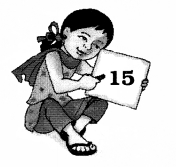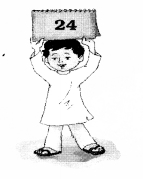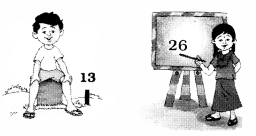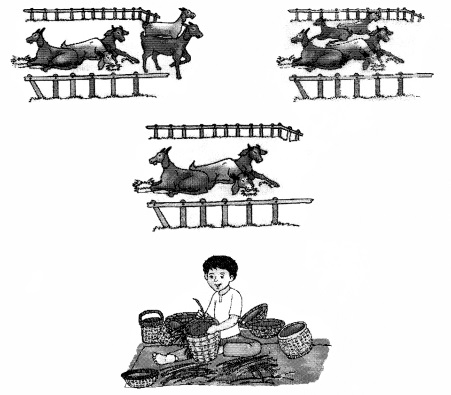NCERT Solutions for Class 1 Maths Chapter 13 How Many includes all the important topics with detailed explanation that aims to help students to understand the concepts better. Students who are preparing for their Class 1 exams must go through NCERT Solutions for Class 1 Maths Chapter 13 How Many. Going through the solutions provided on this page will help you to know how to approach and solve the problems.

Students can also find NCERT intext, exercises and back of chapter questions. Also working on Class 1 Mathematics Chapter 13 How Many NCERT Solutions will be most helpful to the students to solve their Homeworks and Assignments on time. Students can also download NCERT Solutions for Class 1 Maths Chapter 13 How Many PDF to access them even in offline mode.

Board: CBSE
Textbook: NCERT
Class: Class 1
Subject: Maths (Math Magic)
Chapter Name: How Many
Number of Questions – 7

## NCERT Solutions for Class 1 Maths Chapter 13 How Many

NCERT Solutions for Class 1 Maths Chapter 13 How Many are been solved by top teachers of LearnCBSE.in. All the solutions given in this page are solved based on CBSE Syllabus & NCERT guidelines. So before getting into the details of CBSE NCERT Solutions for Class 1 Math Magic Chapter 13 How Many let’s have an overview of list of topics and sub topics under this chapter.

• Write the Number of Sticks
• How much will the bananas cost?
• Which is the bigger number?
• Develop a story on the pictures and narrate in the class.

NCERT Textbook Page 130

Question 1.
Write the number of sticks :Question 2.Question 3.
How much will the bananas cost?Question 4.
Give number name.Fifteen

Question 5.
How many tens?Question 6.
Which is the bigger number?